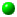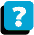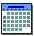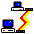Module 5.2 Notes "Confidence Interval Estimation and Hypothesis Testing for a Proportion"

 Index to Module 5 Notes5.1: Simple, Joint, Marginal and Conditional Probabilities5.2: Confidence Interval and Hypothesis Testing for a Proportion5.3: Multiple Sample Tests with Categorical Data

Module Notes 5.1 covered the basics of descriptive statistics for analysis of categorical data.

Pause and Reflect

We describe data for categorical variables by counting the observations of interest within the class or event divisions of the categorical variable. We can convert the count into a probability (also called long term relative frequency, proportion or percent) by dividing the number of observations in the event of interest by the total observations from all the events in the sample space. When we are interested in studying the relationship between two categorical variables, we can exam joint and marginal events; and joint, marginal and conditional probabilities as well.

When the data collected for a categorical variable represents a sample, from a survey for example, we are often interested in making an inference from the sample to the population. Recall that the tools of inferential statistics are confidence intervals and tests of hypothesis.

One of the most popular applications of confidence intervals for the population proportion is the manner in which public opinion polls are reported. Often following a major political news story, the media sponsors public opinion polls via the telephone. Typically 1,000 to 2,000 registered voters are called across the country and asked questions like "do you agree or disagree with _____ on ______." At the 11p.m. news, we then hear something like "55% of registered votes in the US agree with ___ on ____." The really good polling organizations then follow the statement with, "the margin of error is 3%." Guess what - that's a confidence interval for a proportion!!

Confidence Interval Estimation for the Proportion

We are interested in using a sample proportion to estimate the population proportion. We do this similar to any confidence interval.

Pause and Reflect
A confidence interval for the true population parameter being estimated is the sample estimate of that parameter plus/minus the sampling error. The sampling error is a function of the confidence level, the variation in the data, and the sample size.

The population parameters of interest up to now were the mean, median and variance of numerical variables, and the slope and dependent variable of regression relationships. The population parameter of interest to us now is the population proportion, p. The sample statistic used to estimate p is the sample proportion, ps. The sample proportion is determined by dividing the count of observations in the event class of interest by the total observations in all event classes in the sample space.

For example, suppose we surveyed 1,000 shoppers at Kmart and asked them to rate their shopping experience as excellent, good or poor. Here, there are three event classes in the categorical variable called "rating." Further suppose that of the 1,000 shoppers, 272 rated the experience as excellent.

Equation 5.2.1 shows the calculation for the sample proportion:

Eq. 5.2.1: ps = X / n = 272 / 1,000 = 0.272 or 27.2%
where X = the count of observations within the event class of interest

Technically, when a categorical variable of interest has only two outcomes, such as success or failure, male or female, success or failure, excellent rating or not excellent rating, that categorical variable follows a distribution called the binomial distribution. Any sampling distribution used in estimating a population parameter has a mean and a standard error. For a sampling distribution that follows the binomial distribution, the mean is ps and the standard error is:

Eq. 5.1.2: Standard Error of ps = Square Root [ ps * ( 1-ps) / n] =
Sq Rt [ 0.272 * (1 - 0.272) / 1,000] = 0.014

As long as the sample size is assumed to be sufficiently large, the binomial distribution is approximated by the normal distribution which means that we can use the Z statistic to form the sampling error portion of the confidence interval. The test for "large" is that both nps and n(1-ps) are at least 5. For this sample, we meet the "large" test since nps = 1,000 * 0.272 = 272 and n * (1-ps) = 1,000 * (1- 0.272) = 728: 272 and 728 are both greater than 5.

Now we can put together the confidence interval for the population proportion. The 95% confidence interval for the proportion is:

Eq. 5.2.3: 95% Confidence Interval for the True Population Proportion:
= ps + Z * Std Error of ps
= ps + 1.96 * Sq Rt [ ps * (1 - ps) / n ]
= 0.272 + 1.96 * Sq Rt [0.272 * (1 - 0.272) / 1,000]
= 0.272 + 1.96 * 0.14
= 0.272 + 0.028
or 0.244 < p < 0.300

The interpretation: we are 95% confident that between 24.4% and 30.0% of this population rate the shopping experience at Kmart as excellent. Although the math is fairly easy for a confidence interval for a proportion, we can set up an Excel Worksheet template as shown in Worksheet 5.2.1:

Worksheet 5.2.1
 Row 1 Col I J K 2 Confidence Interval for Proportion 3 n 1000 4 Successes 272 5 Failures 728 = J3 - J2 6 Confidence Level 0.95 7 8 Sample Proportion 0.272 = J4 / J3 9 Z 1.96 = NORMSINV(0.5+(J6/2)) 10 Standard Error 0.014 = SQRT((J8*(1-J8))/J3) 11 Half-Width of C.I. 0.028 = J9 * J10 12 Lower Limit of C.I. 0.244 = J8 - J11 13 Upper Limit of C.I. 0.300 = J8 + J11

Do you remember how the analyst can make the sampling error portion of the confidence interval tighter (smaller)? That's right - increase the sample size. What happens when we use a sample size of 10,000:

Eq. 5.2.4: 95% Confidence Interval for the True Population Proportion:
= ps + Z * Std Error of ps
= ps + 1.96 * Sq Rt [ ps * (1 - ps) / n ]
= 0.272 + 1.96 * Sq Rt [ 0.272 * (1 - 0.272) / 10,000 ]
= 0.272 + 1.96 * 0.0045
= 0.272 + 0.009
or 0.263 < p < 0.281

We have certainly come closer to capturing the true population proportion - of course we have, we sampled 10,000 people! That can be expensive. So, most of the time, you hear polling companies say, the sampling error (they actually say margin of error most of the time) is + 3%. Exactly how large a sample do you need to get a margin of error of exactly 3%?

Computational Formula for the Sample Size for Estimating the Proportion
To find this out, we solve the following sample size formula:

Eq. 5.2.5: n = [ Z2 * p * (1-p) ] / e2
where e = the desired sampling error,
and p = true population proportion.

Often (most of the time) we do not know the true population proportion - if we did, we would not need to find the confidence interval! So, a conservative approach is to let p = 0.50, which results in the largest value for n. If we want to be 95% confident, and want a sampling error of 3%, the formula becomes:

Eq. 5.2.6: n = [1.96 * (0.50) * (1 - 0.50) ] / 0.03 = 1,067.

As I stated earlier, this is the figure most often used by polling companies. Of course, to get 1,067 responses, you have to call more than 1,067 citizens to account for the non responses.

That is how one estimates a population proportion using a confidence interval. Let me close by noting what to do if you have a finite population.

Finite Population Correction Factor
Most populations we deal with are really finite - not infinite. Technically speaking, the confidence interval formula for estimating the population proportion applies to infinite populations. However, in reality, the formula works just fine as long as the population is not too small compared to the sample size. The rule of thumb is that as long as the sample size is less than 5% of the population size (Sincich, p. 412), there is no need to apply the finite population correction factor (Levine, 2000).

For example. What if the Kmart store used in these notes is located in Cape Coral, with an approximate population of 100,000. If the sample size is 1,000, then the ratio of sample size to population is 1,000/100,000 or 1% which passes the above rule of thumb. On the other hand, if you planned to survey the 100 winter residents of Upper Captiva on some issue, and your sample size was 50, then the ratio of sample size to population size is 50% and the finite population correction factor should be applied.

Any time we have to adjust for a finite population, the confidence interval formula becomes:

Eq. 5.2.7: 95% Confidence Interval for the True Population Proportion with Finite Population Correction Factor:

= ps + Z * Std Error of ps * Sq Rt [ (N - n) / (N - 1)]
= ps + 1.96 * Sq Rt [ps * (1 - ps) / n ] * Sq Rt [ (N - n) / (N - 1)]

The sample size formula becomes:

Eq. 5.2.8: n = no * N / [ no + (N - 1 ) ] where no is the sample size determined from Eq. 5.2.5

The other tool of inferential statistics is the test of hypothesis, this time for the true population proportion.

Test of Hypothesis for the Proportion

Suppose we wanted to test if the true population proportion of Kmart shoppers rating the shopping experience at Kmart as excellent was equal to 32% versus not equal to 32%. We would proceed as with any hypothesis test, starting with the hypotheses:

H0: p = 0.32 (null hypothesis)
Ha: p =/= 0.32 (alternative hypothesis)

We would gather our data, compute the sample statistics, and then the test statistic. Using the above example, the sample proportion for a sample size of 1,000 shoppers came out to be 0.272 or 27.2%. The test statistic for large sample test of hypothesis for the population proportion is the Z:

Eq. 5.2.9: Z = (ps - p) / Sq Rt [ p * (1 - p) / n ] where p is hypothesized p.
Z = (0.272 - 0.32 ) / Sq Rt [ 0.32 * (1- 0.32) / 1000] = - 3.254

The probability of us getting a sample proportion of 27.2%, given a hypothesized proportion of 32% is the p-value, obtained by the Excel function =NORMSDIST(-3.254). This function returns a p-value of 0.0005. Since this is a two tailed probability, and since the =NORMSDIST function returns a lower tail p-value, we have to multiply the p-value by 2 to get the full p-value. This gives 0.001.

The conclusion is that since the p-value is less than a specified value of alpha of 0.05, we reject the null hypothesis and conclude that the population proportion is not equal to 32%. From the sample proportion, we would further conclude that the proportion is less than 32%, and from the confidence interval computed in Equation 5.2.3, we are confident that the true proportion lies between 24.4% and 30.0%. Note that the confidence interval confirms the result of the test of hypothesis, which will always be the case with a not equal, two-tailed alternative hypothesis.

Worksheet 5.2.2 provides a template for test of hypothesis for a proportion.

Worksheet 5.2.2
 1 Column R S T 2 Hypothesis Test for the Proportion 3 n 1000 4 Successes 272 5 Sample Proportion 0.272 = S4 / S3 6 Null Hypothesis p = 0.32 7 Standard Error 0.015 = SQRT((S6*(1-S6))/S3) 8 Alpha 0.050 9 Z Test Statistic -3.254 = (S5 - S6) / S7 10 p-value (1-tail) 0.0006 = (1-NORMSDIST(ABS(S9))) 11 p-value (2-tail) 0.0011 = 2 * S10 12

This template is designed so that the user need only insert information in cells S3, S4, S6 and S8. The computation formulas in the remaining cells in Column S are shown as notes in Column T.

Material covered in this module note can be used to answer questions one and two of the Airline Satisfaction Survey Assignment in Main Module 5 Overview.

Our last Module Note 5.3 covers methods of comparing multiple samples of categorical data.

References:

Anderson, D., Sweeney, D., & Williams, T. (2001). Contemporary Business Statistics with Microsoft Excel. Cincinnati, OH: South-Western, Chapter 7 (Section 7.6) and Chapter 8 (Section 8.4).

Levine, D., Berenson, M. & Stephan, D. (1999). Statistics for Managers Using Microsoft Excel (2nd. ed.). Upper Saddle River, NJ: Prentice-Hall, Chapters 7 and 8.

Mason, R., Lind, D. & Marchal, W. (1999). Statistical Techniques in Business and Economics (10th. ed.). Boston: Irwin McGraw Hill, Chapters 8 and 9.

Sincich, T. (1992). Business Statistics by Example (4th ed.). New York: Dellen/ Macmillan Publishing CompanyAbout the CourseModule ScheduleWebBoard